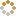﻿ N-B-U-T Online Judge ::  Discount
• ###  Discount

• 时间限制: 1000 ms　内存限制: 65535 K
• 问题描述
• You just need to calculate the price of something after discount.
• 输入
• This problem has several cases. Each case contains a number P (0.00 < P < 888888.00), means the price. And then follows a number D (1.00 <= D <= 9.99), means the discount.
• 输出
• For each case, you just need to calculate the price after discount.
• 样例输入
• ```10.00 8.80
```
• 样例输出
• ```8.80
```
• 提示
• `无`
• 来源
• `XadillaX`
• 操作
﻿